Pages created and updated by Terry Sturtevant Date Posted: June 5, 2017

# Maple Tutorial: Phasors and AC Circuits

Maple is a commercial program. It is a computer algebra system (CAS) like Maxima or Mathematica.
There are various tutorials out there on how to use Maple; this one is designed to focus on its use for AC circuit analysis; i.e. the use of complex numbers for AC analysis with capacitors and inductors.

## Sample AC Circuit

A basic example of the use of phasors is the investigation of simple series and parallel LC circuits. For ideal components,
ZL= I ω L
and
ZC= 1/ (I ω C )
Here are simple series and parallel LC circuits: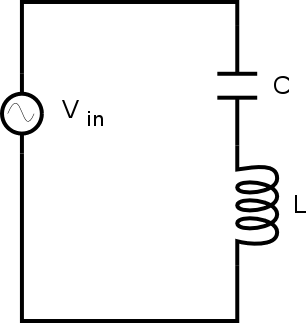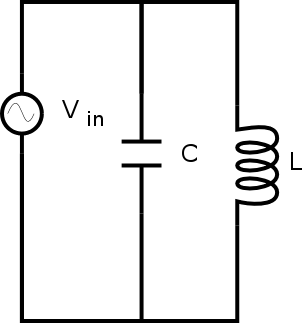A computer algebra system can be very useful for analyzing circuits like this.

5. ### Entire Session Transcript

1. Opening Maple:
• Open Maple from the menu or the desktop icon.

2. Defining Impedances
• It is quite useful to not have to specify component values at the beginning, because the results can be determined algebraically once, after which it is only necessary to substitue in specific quantities.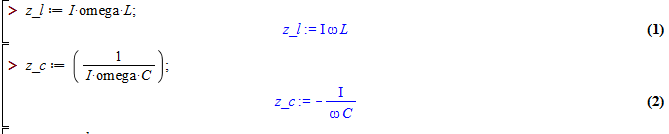3. Series Circuit Properties
• The impedance of an LC circuit is easy to check in 3 specific situations;
the high frequency limit,
the DC frequency limit,
the resonant frequency, where ω = 1/√ LC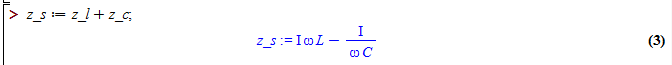Limits are useful for testing the high frequency case: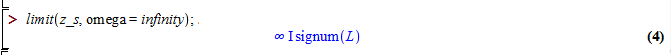Note in the high frequency case, where ω goes to infinity, the mathematical result depends on the sign of L; since L can only be positive physically, then this gives the expected result.
Limits can also be used for the low frequency case: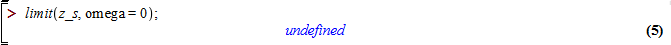Finding the value at the resonant frequency can be done by using the limit as well: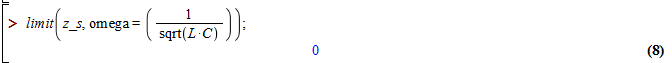It can also be found by substitution, as shown in the following: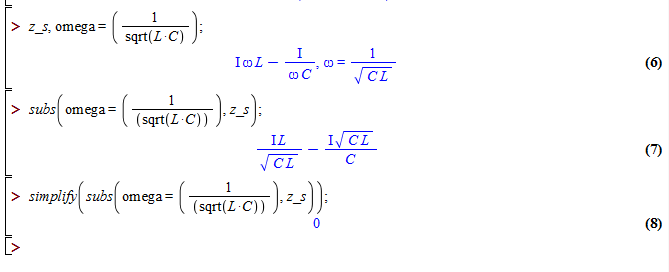Note that without using the simplify function, the fact that the result is zero is not immediately apparent.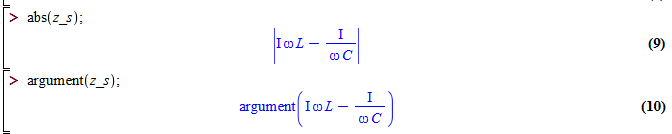It's not clear from the above whether the argument function is works, but a further step clarifies it: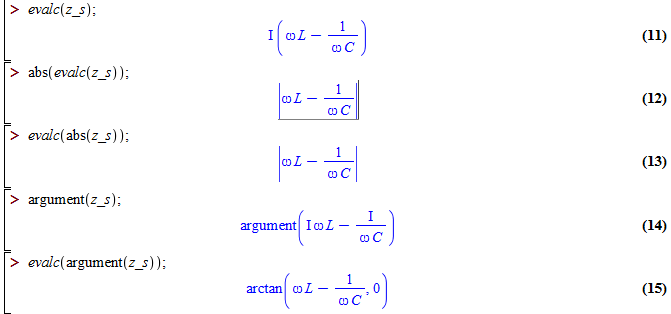The evalc function is for evaluating complex numbers, and taking the absolute value gives the magnitude. (Note the order of abs and evalc doesn't matter.)
4. Parallel Circuit Properties

•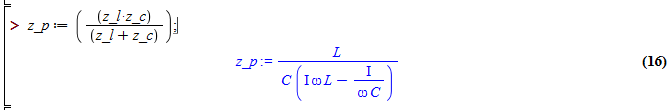The high and low frequency cases can be tested as before: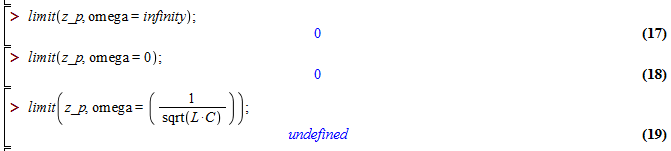Since the impedance at the resonant frequency is undefined, the limit has to be used.

5. Entire Session Transcript: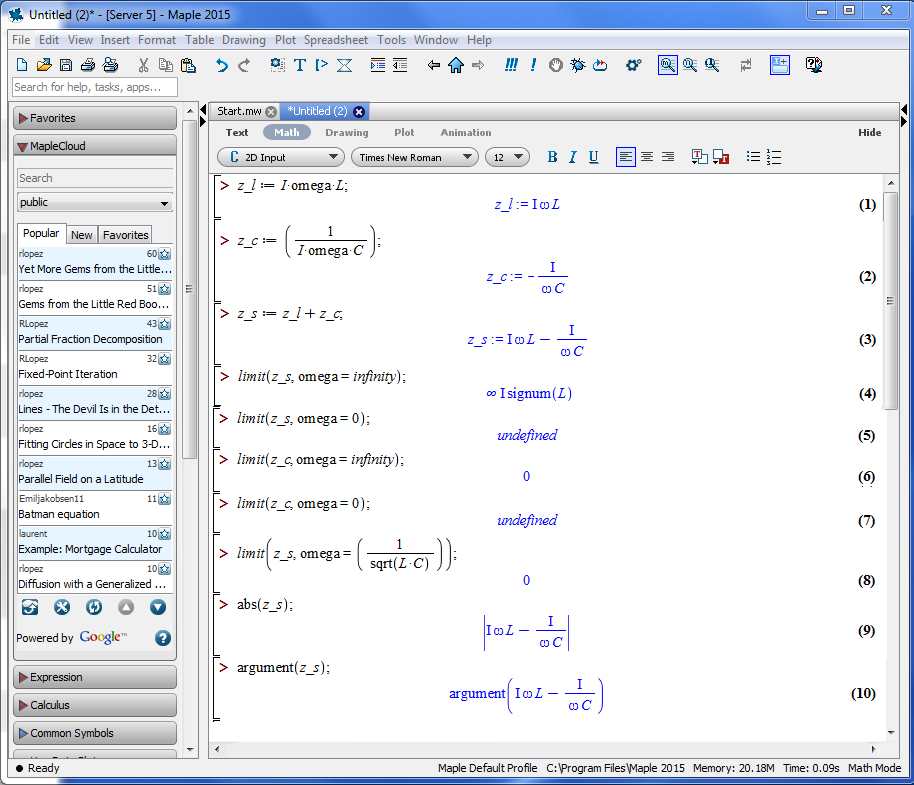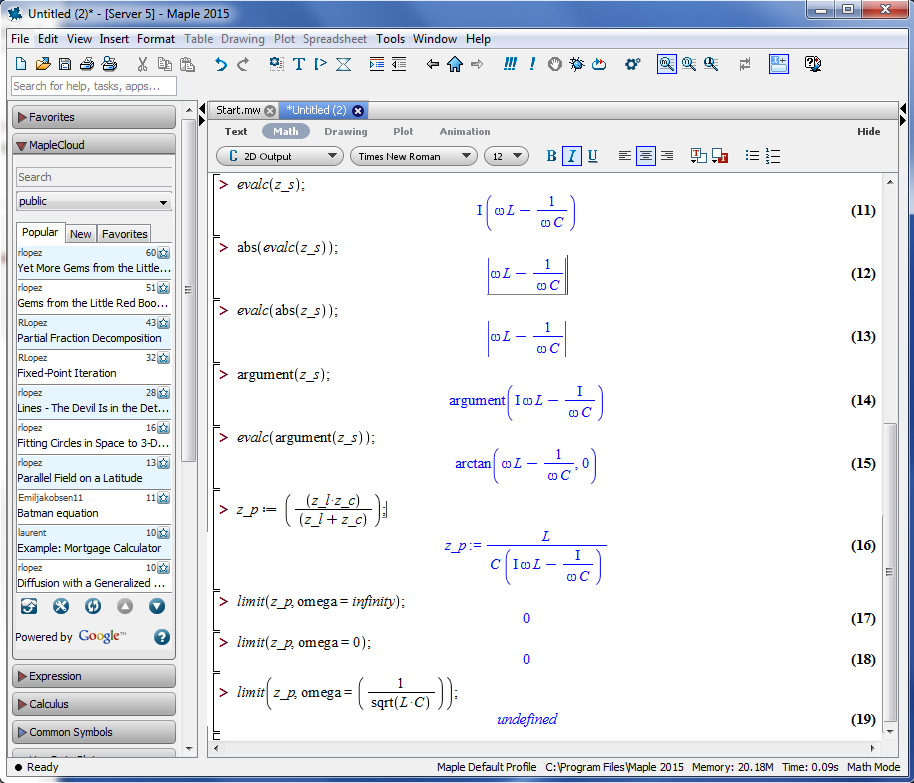Information on this site which is produced by Terry Sturtevant is licensed under a

## Resources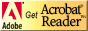If you need to update a browser, you might try Firefox which is
• free
• open source
• available for several platformsSince this page uses cascading style sheets for its layout, it will look best with a browser which supports the specifications as fully as possible.

If you are looking for an office package, with a word processor, spreadsheet, etc., you might try LibreOffice which is
• free
• open source
• available for several platforms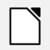Go to the main page for the Department of Physics and Computer Science.

Wilfrid Laurier University